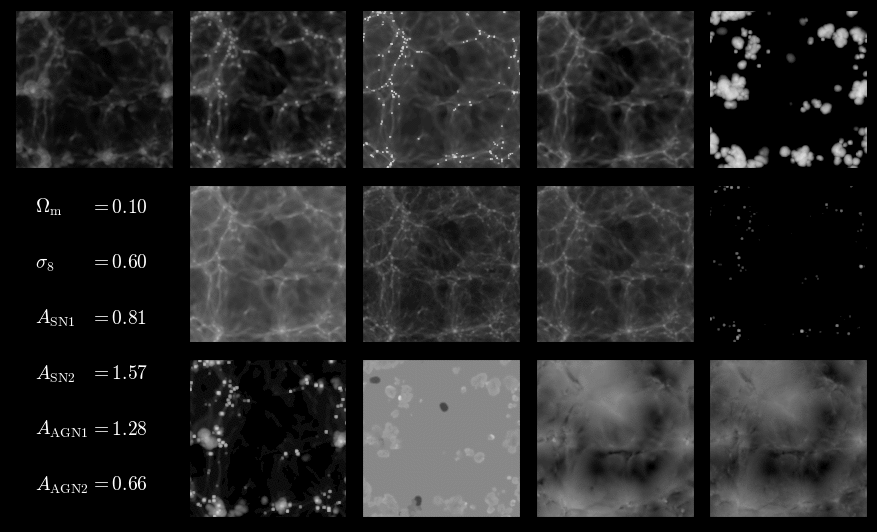# CAMELS Multifield Dataset (CMD)CMD is a publicly available collection of hundreds of thousands 2D maps and 3D grids containing different properties of the gas, dark matter, and stars from more than 2,000 different universes. The data has been generated from thousands of state-of-the-art (magneto-)hydrodynamic and gravity-only N-body simulations from the CAMELS project.Examples of 2D maps from CMD. From top-left to bottom right: gas temperature, gas pression, neutral hydrogen mass, electron number, metallicity, gas mass, dark matter mass, total mass, stellar mass, magnetic fields, magnesium over iron, gas velocity, and dark matter velocity. Each map is characterized by the value of the cosmological parameters ($$\Omega_{\rm m}$$ and $$\sigma_8$$) and the astrophysical parameters ($$A_{\rm SN1}$$, $$A_{\rm SN2}$$, $$A_{\rm AGN1}$$, and $$A_{\rm AGN2}$$).

Each 2D map and 3D grid has a set of labels, or parameters, associated to it. Understanding the relation between the data and the labels will help cosmologists to decipher the mysteries of our own Universe.